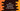# Write a Java program to find current resolution of the Screen## Java program to find current Screen Resolution :

In this tutorial, we will learn how to find the Screen resolution of your system. We will use ‘java.awt’ package to calculate the values.

1. Using ‘Toolkit’ class, get the screen size using ‘Toolkit.getDefaultToolkit().getScreenSize()’ method.
2. getScreenSize()’ gets the size of the primary display if multiple screens are added.
3. The return value is of type ‘Dimension’
4. We can have the ‘height’ and ‘width’ using ‘getHeight()’ and ‘getWidth()’ methods.
5. The return values of ‘width’ and ‘height’ is in pixel.

### Example program :

``````import java.awt.*;

public class Main {

public static void main(String[] args) {

Dimension size = Toolkit.getDefaultToolkit().getScreenSize();
short width = (short) size.getWidth();
short height = (short) size.getHeight();

System.out.println("Current Screen resolution : " + "w : " + width + " h : " + height);

}
}``````

### Output :

``Current Screen resolution : w : 1366 h : 768``